Home | | Structural Analysis I | Solved Problems: Slope Deflection Method- Structural Analysis

# Solved Problems: Slope Deflection Method- Structural Analysis

Civil - Structural Analysis - Slope Deflection Method

SLOPE DEFLECTION METHOD

(1). A beam ABC, 10m long, fixed at ends A and B is continuous over joint B and is loaded as shown in Fig. Using the slope deflection method, compute the end moments and plot the bending moment diagram. Also, sketch the deflected shape of the beam. The beam has constant EI for both the spans.

Solution.

(a)  Fixed end moments

Treating each span as a fixed beam, the fixed end moments are as follows:(b) Slope deflection equations

The end rotations qA and qC are zero since the beam is fixed at A and C. hence there is only one unknown, qB. the ends do not settle and hence dfor each span is zero. Let us assume qB to be positive. The result will indicate the correct sign. The slope deflection equations are as follows:

For span AB,For span BC,(c)  Equilibrium equation

Since there is only one un known, i.e. qB, one equilibrium equation is sufficient. For the joint B, we have

MBA + MBC = 0

\(0.8 EI qB + 3.6) + (0.8 EI qB + 5.0) = 0 1.6 EI qB = 1.4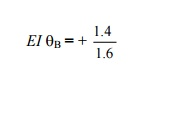The plus sign indicates that qB is positive (i.e. rotation of tangent at B is clockwise).

(d) Final moments

Substituting the values of EI qB in Eqa. (1) to (4), we get(2) A beam ABC, 10m long, hinged at ends A and B is continuous over joint B and is loaded as shown in Fig. Using the slope deflection method, compute the end moments and plot the bending moment diagram. Also, sketch the deflected shape of the beam. The beam has constant EI for both the spans.

SOLUTIONS

(a) Fixed end moments

These are the same as calculated in the previous problem:

MFAB = -2.4 KN-m ; MFBA = +3.6 KN-m

MFBC = -5.0 KN-m ; MFCB = +5.0 KN-m

(b) Slope deflection equations.(c) Equilibrium equations

Since end A is freely supported,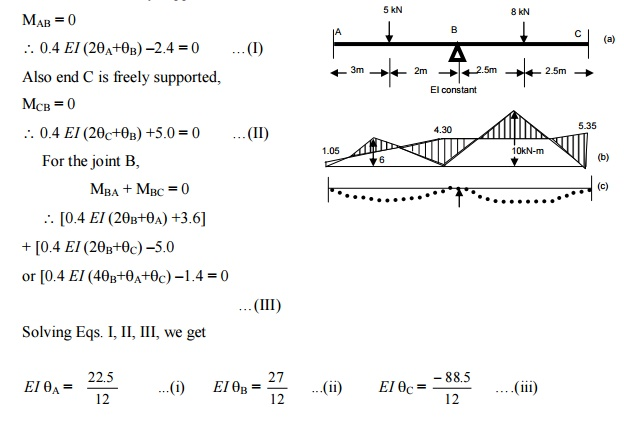(d) Final moments : Substituting the values of EI qA and EI qB inEq. (2), we getThe bending moment diagram and the deflected shape of the beam are shown in the Fig. Note. The beam is statically indeterminate to single degree only. This problem has also been solved by the moment distribution method (example 10.2) treating the moment at B as unknown. However, in the4 slope- deflection method, the slope or rotations are taken as unknowns, and due to this the problem involves three unknown rotations qA, qB and qC. hence the method of slope deflection is not recommended for such a problem.

(3) A continuous beam ABCD consists of three spans and is loaded as shown in fig. ends A and D are fixed. Determine the bending moments at the supports and plot the bending moment diagram.

a)Fixed end moments(b) Slope deflection equation

qA and qD are zero since ends A and D are fixed.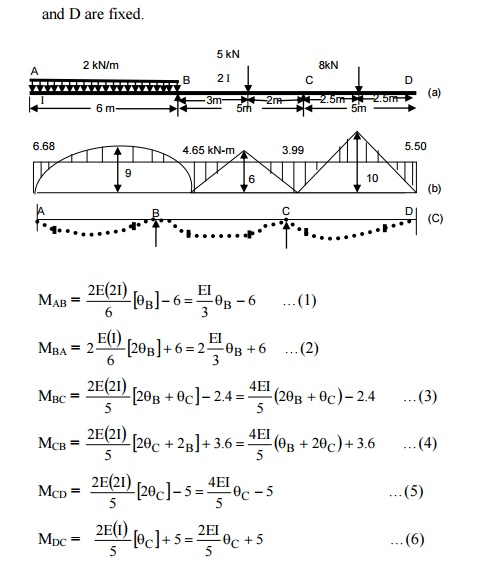(c)  Equilibrium equations

At join B, MBA + MBC = 0At join C, MCB + MCD = 0

From (I) and (II), we get  EI qB = -2.03 kN-m and EI qC =  + 1.26kN-m

(d) Final moments

Substituting this values in Eqs. (1) to (6), we get

The bending moment diagram and the deflected shape are shown in Figure.4) A continuous beam ABC is supported on an elastic column BD and is loaded as shown in figure . Treating joint B as rigid, analyze the frame and plot the bending moment diagram and the deflected shape of the structure.

(a)Fixed end momentsMFBD = MFDB = 0

(b)Slope deflection equations.

The slopes qA and qD are zero since ends A and D are fixed.

For span AB(c) Equilibrium equations

At join B, MBA + MBC + MBD = 0(d) Final moments

Substituting this values in Eqs. (1) to (6), we get

The bending moment diagram and the deflected shape are shown in Figure.(5) Analyze the rigid frame shown in figure

(a) Fixed end moments(b) Slope deflection equations.(c)  Equilibrium equations

For the equilibrium joint B,  MBA + MBD + MBC = 0

\(2EIqB + 2.67) + (EIqB -2) + (-4) = 0

3EIqB = 3.33

EIqB = 1.11

(d) Final moments

Substituting this value of EIqB in Eqs. (1) to (4), we getThe bending moment diagram and

The deflected shapes are shown in Figure.

(6) A portal frame ABCD is fixed at A and D, and has rigid joints at B and C. The column AB is 3m long. The beam BC is 2m long, and is loaded with uniformly distributed load of intensity 6 kN/m. The moment of inertia is 2.1 and that of BC and CD is I (Fig). Plot B.M. diagram and sketch the deflected shape of the frame.

(a) Fixed end moments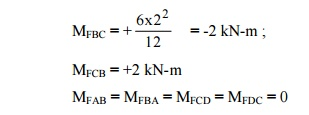Let the joints B and C move horizontally by d

(b)Slope deflection equations.(c)      Equilibrium equations.

At joint B,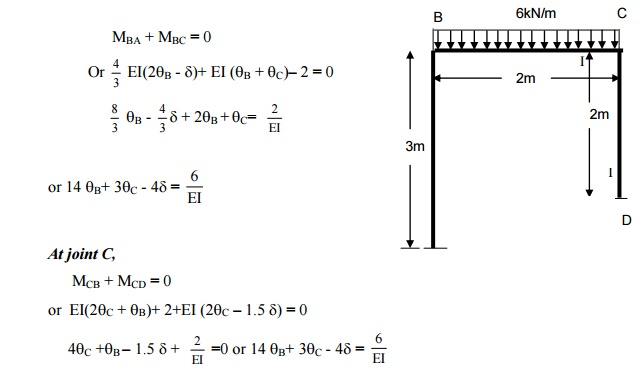d )Shear equation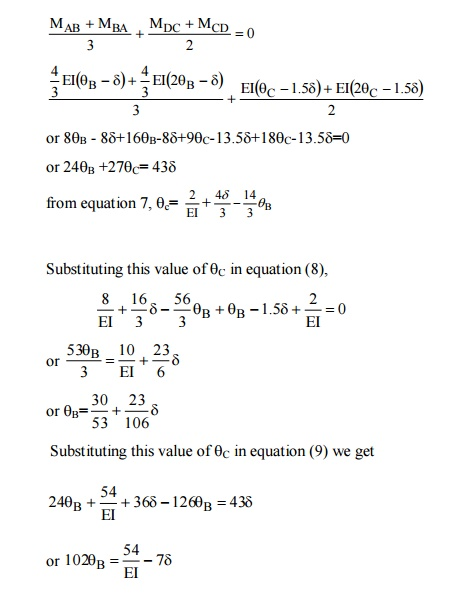e) Final moments(7) A portal frame ABCD is hinged at A and fixed at D and has stiff joints at B and C. the loading is as shown in figure. Draw the bending moment diagram and deflected shape of the frame.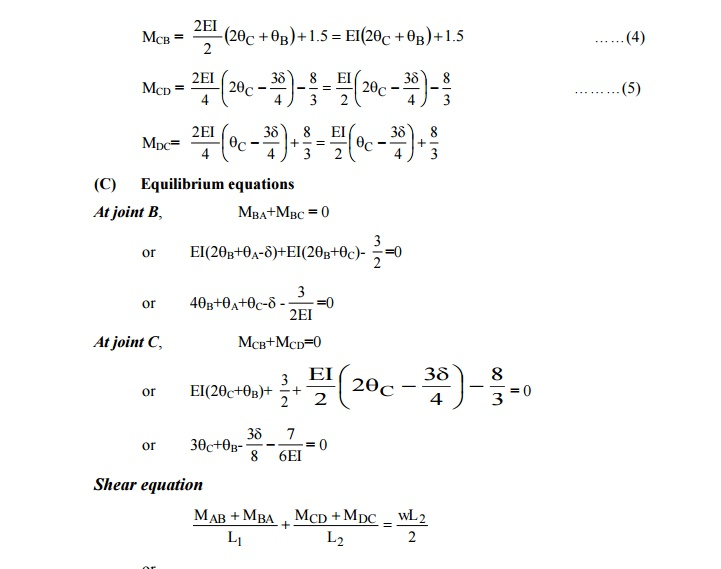Study Material, Lecturing Notes, Assignment, Reference, Wiki description explanation, brief detail
Civil : Structural Analysis : Slope Deflection Method : Solved Problems: Slope Deflection Method- Structural Analysis |